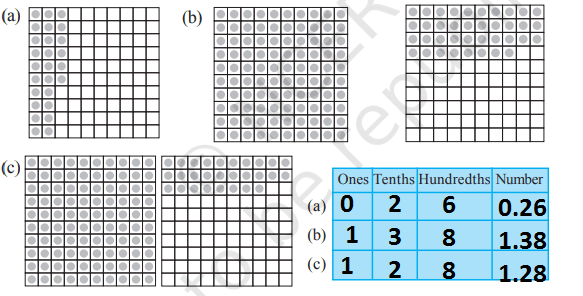# NCERT Class 6 Mathematics Eighth Chapter Decimals Exercise 8.2 Solutions

## EXERCISE 8.2

(1) Complete the table with the help of these boxes and use decimals to write the number.(2) Write the numbers given in the following place value table in decimal form.(a) 3.25

(b) 102.63

(c) 30.025

(d) 211.902

(e) 12.241

(3) Write the following decimals in the place value table.(4) Write each of the following as decimals.In case you are missed :- Previous Chapter Exercise Solution

(5) Write each of the following decimals in words.

(a) 0.03 = Zero point zero three

(b) 1.20 = One point two zero

(c) 108.56 = One hundred eight point five six

(d) 10.07 = Ten point zero seven

(e) 0.032 = Zero point zero three two

(f) 5.008 = Five point zero zero eigth

(6) Between which two numbers in tenths place on the number line does each of the given number lie?

(a) 0.06 = 0 and 0.1

(b) 0.45 = 0.4 and 0.5

(c) 0.19 = 0.1 and 0.2

(d) 0.66 = 0.6 and 0.7

(e) 0.92 = 0.9 and 1.0

(f) 0.57 = 0.5 and 0.6

(7) Write as fractions in lowest terms.In case you are missed :- Next Chapter Exercise Solution

Updated: April 14, 2023 — 2:46 pm